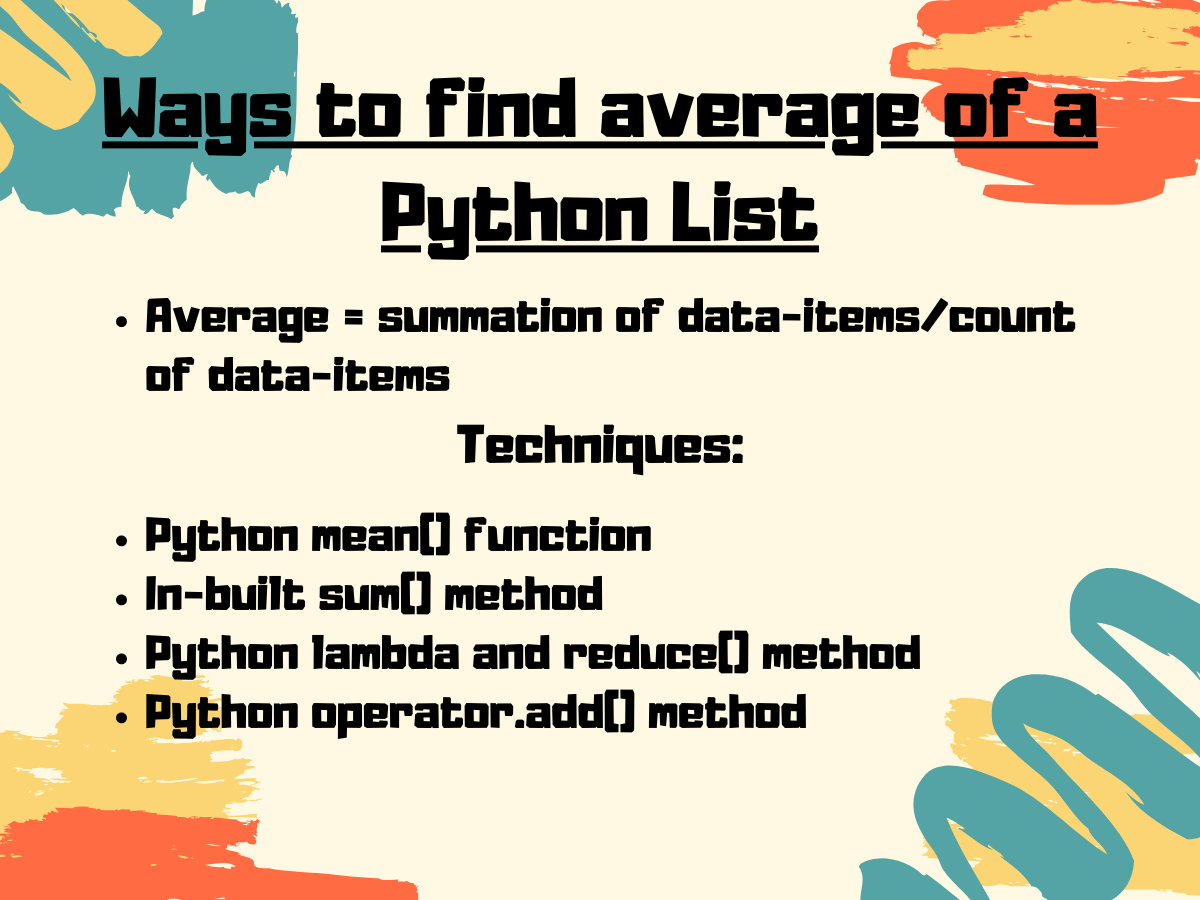# 5 Ways to Find The Average of a List in Python

Published on August 3, 2022By Safa MulaniWhile we believe that this content benefits our community, we have not yet thoroughly reviewed it. If you have any suggestions for improvements, please let us know by clicking the “report an issue“ button at the bottom of the tutorial.

Hi Folks! In this article, we will have a look at the various ways to find the average of a list in a Python List.

In general, an average is a value that represents a whole set of data items or elements.

Formula: Average = summation of numbers/total count.

## Techniques to find the average of a list in Python

Either of the following techniques can be used to calculate the average/mean of a list in Python:

• Python mean() function
• In-built sum() method
• Python lambda and reduce() method

### 1. Python mean() function

Python 3 has `statistics module` which contains an in-built function to calculate the mean or average of numbers. The `statistics.mean() function` is used to calculate the mean/average of input values or data set.

The mean() function accepts the list, tuple or data-set containing numeric values as a parameter and returns the average of the data-items.

Syntax:

``````mean(data-set/input-values)
``````

Example:

``````from statistics import mean

inp_lst = [12, 45, 78, 36, 45, 237.11, -1, 88]
list_avg = mean(inp_lst)

print("Average value of the list:\n")
print(list_avg)
print("Average value of the list with precision upto 3 decimal value:\n")
print(round(list_avg,3))

``````

In the above snippet of code, we have used `statistics.round()` method to round off the output average up to a particular decimal value.

Syntax:

``````statistics.round(value, precision value)
``````

Output:

``````Average value of the list:

67.51375
Average value of the list with precision upto 3 decimal value:

67.514
``````

### 2. Using Python sum() function

Python `statistics.sum()`function can also be used to find the average of data values in Python list.

The `statistics.len()` function is used to calculate the length of the list i.e. the count of data items present in the list.

Syntax:

``````len(input-list)
``````

Further, `statistics.sum()` function is used to calculate the sum of all the data items in the list.

Syntax:

``````sum(input-list)
``````

Note: average = (sum)/(count).

Example:

``````from statistics import mean

inp_lst = [12, 45, 78, 36, 45, 237.11, -1, 88]

sum_lst = sum(inp_lst)

lst_avg = sum_lst/len(inp_lst)
print("Average value of the list:\n")
print(lst_avg)
print("Average value of the list with precision upto 3 decimal value:\n")
print(round(lst_avg,3))
``````

Output:

``````Average value of the list:

67.51375
Average value of the list with precision upto 3 decimal value:

67.514

``````

### 3. Using Python reduce() and lambda method

We can use Python reduce() function along with the lambda() function.

Python reduce() function: The `reduce() function` is basically used to apply a particular(input) function to the set of elements passed to the function.

Syntax:

``````reduce(function,input-list/sequence)
``````
• Initially, the reduce() function applies the passed function to the first two consecutive elements and returns the result.
• Further, we apply the same function to the result obtained in the previous step and the element succeeding the second element.
• This process continues until it reaches the end of the list.
• Finally, the result is returned to the terminal/screen as output.

Python lambda() function: The `lambda() function` is used to build and form Anonymous functions i.e. function without a name or signature.

Syntax:

``````lambda arguments:function
``````

Example:

``````from functools import reduce

inp_lst = [12, 45, 78, 36, 45, 237.11, -1, 88]

lst_len= len(inp_lst)

lst_avg = reduce(lambda x, y: x + y, inp_lst) /lst_len
print("Average value of the list:\n")
print(lst_avg)
print("Average value of the list with precision upto 3 decimal value:\n")
print(round(lst_avg,3))

``````

Output:

``````Average value of the list:

67.51375
Average value of the list with precision upto 3 decimal value:

67.514

``````

### 4. Python operator.add() function to find the average of a list

The Python operator module contains various functions to perform basic calculations and operations efficiently.

The `operator.add()` function can be used to calculate the summation of all the data values present in the list with the help of Python reduce() function.

Syntax:

``````operator.add(value1, value2)
``````

Note: average = (sum)/(length or count of elements)

Example:

``````from functools import reduce
import operator
inp_lst = [12, 45, 78, 36, 45, 237.11, -1, 88]

lst_len = len(inp_lst)

print("Average value of the list:\n")
print(lst_avg)
print("Average value of the list with precision upto 3 decimal value:\n")
print(round(lst_avg,3))
``````

Output:

``````Average value of the list:

67.51375
Average value of the list with precision upto 3 decimal value:

67.514
``````

### 5. NumPy average() method to calculate the average of a list in Python

Python’s NumPy module has an in-built function to calculate the average/mean of the data items present in the data set or list.

The `numpy.average()` method is used to calculate the average of the input list.

Example:

``````import numpy

inp_lst = [12, 45, 78, 36, 45, 237.11, -1, 88]

lst_avg = numpy.average(inp_lst)
print("Average value of the list:\n")
print(lst_avg)
print("Average value of the list with precision upto 3 decimal value:\n")
print(round(lst_avg,3))

``````

Output:

``````Average value of the list:

67.51375
Average value of the list with precision upto 3 decimal value:

67.514
``````

## Conclusion

Thus, in this article, we have unveiled and understood various techniques to find the average of a Python List.

Click below to sign up and get \$200 of credit to try our products over 60 days!

### Popular Topics##### Hollie's Hub for Good

Working on improving health and education, reducing inequality, and spurring economic growth? We’d like to help.##### Become a contributor

You get paid; we donate to tech nonprofits.

##### Welcome to the developer cloud

DigitalOcean makes it simple to launch in the cloud and scale up as you grow – whether you’re running one virtual machine or ten thousand.© 2023 DigitalOcean, LLC.